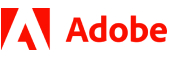New update is available. Click here to update.
Last Updated: 7 Jan, 2021

# Unique Paths

Moderate+19 more companies

## Problem statement#### To traverse in the matrix, you can either move Right or Down at each step. For example in a given point MATRIX[i] [j], you can move to either MATRIX[i + 1][j] or MATRIX[i][j + 1].

##### Input Format:
``````The first line of input contains an integer 'T' representing the number of the test case.

The first and the only line of each test case contains two space-separated integers ‘M’ and ‘N’, denoting the number of rows and number of columns of the matrix respectively.
``````
##### Output Format:
``````For every test case, return a single integer, which is the total number of unique paths for traveling from top-left to bottom-right cells of the matrix.

The output of each test case is printed in a separate line.
``````
##### Note:
``````You don’t have to print anything, it has already been taken care of. Just implement the given function.
``````
##### Constraints:
``````1 ≤ T ≤ 100
1 ≤ M ≤ 15
1 ≤ N ≤ 15

Where ‘M’ is the number of rows and ‘N’ is the number of columns in the matrix.

Time limit: 1 sec
``````## Approaches

### 01 ApproachWe can easily count the total number of paths by making a recursive algorithm.

The steps are as follows:

1. We are given a function UNIQUEPATHS(), which takes two integers ‘M’ and ‘N’ as parameters and returns a single integer. This will be the definition of our recursive function too.
2. As our base condition, we will check if our number of rows (‘M’) or a number of columns (‘N’) is equal to 1. If either one of them is equal to 1, this will mean that it is a 1-D array, and only a single path will be available. Hence we will return 1.
3. If our base condition is not satisfied, we will proceed by calling our recursive function, UNIQUEPATHS() by providing it with the left and upper submatrix. Hence we will return UNIQUEPATHS('M', ‘N’ - 1) + UNIQUEPATHS('M' - 1, ‘N’).
4. After all the recursive calls, the function UNIQUEPATHS() will return the final answer.